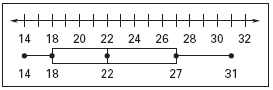# Measures of Variability : Range

The range of a numerical set of data is the difference of the greatest value and the least value. In other words, the largest number minus the smallest number is the range.

If we are given a list of data find the largest and smallest number and subtract them.

14, 15, 13, 11, 17, 12, 18

18 - 11 = 7
Therefore the range is 7.

If you are given a stem and leaf plot:key 2|3 = 23

57 - 23 = 34
Therefore the range is 34

If you are given a box and whisker plot:Min                                                     Max

Max - Min = 31 - 14 = 17
Therefore the range is 17.

Quickly sum it up: Regardless of the way the data is given the range is the largest number minus the smallest number.

 Related Links: Math algebra Parts of An Expression Positive and Negative Integer Exponents

To link to this Measures of Variability : Range page, copy the following code to your site: